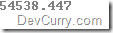# Chain Extension Methods in .NET

 Tweet

Extension methods enable you to add methods to existing types without creating a new derived type, recompiling, or otherwise modifying the original type. You can even chain Extension Methods.

Here’s an example:

`class Program{    static void Main(string[] args)    {        decimal num = 233.53468M;        // chaining extension methods        decimal result = num.DoSquare().DoRound();        Console.WriteLine(result);        Console.ReadLine();    }}public static class MathEasy{    public static decimal DoSquare(this decimal i)        {        // square a number        return i * i;    }    public static decimal DoRound(this decimal d)        {        // Round to three decimal places        return Math.Round(d, 3);    }}`

As you can see, we are chaining extension methods

`decimal result = num.DoSquare().DoRound();`

OUTPUT